## Pages

### Electromagnetism - Detailed Explanation

Why do we call the subject electromagnetism?
For stationary charges Coulomb’s law holds. But it is not true, when charges are moving the electrical forces depends also on motion of charges in a complicated way. One part of the force between moving charges we call the magnetic force. It is really one aspect of electric effect. This is why we call the subject Electromagnetism.

What is Field?
A “FIELD” is any physical quantity which takes on different values at different points in spaces.

Example: -     Temperature --------  a scalar field T(x,y,z).
Velocity of a flowing fluid is vector field.

The relationship between the values of field at one point and the values at a nearby point are very simple With only a few relationship in form of differential equations we can describe fields completely. It is in terms of such equations that laws of electrodynamics are most simply written.

Characteristics of vector field
There are two mathematically important properties of a vector field which we use in description of laws of electricity from field point of view.
·       The flux of vector field.
·       The circulation of vector field.
For an arbitrary closed surface, the next outward flow or flux is the average outward normal component of vector field times the area of surface.
Flux = (average normal component)*(surface area)

The second property of vector field has to do with a line rather than a surface. If there is a net rational motion around some loop then vector field is said to be circulating.

For any vector field the circulation around any imaginary closed curve is defined as average tangential component of vector multiplied by circumference of loop.
Circulation = (average tangential component)*(distance around)
The properties flux and circulation can describe as laws of electricity and magnetism at once.

The laws of electromagnetism
The first law of electromagnetism describes the “flux” of electric field.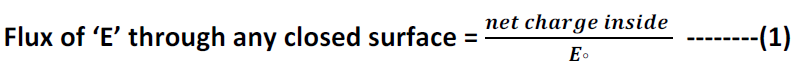If we have an arbitrary stationary curve in space and measure circulation of electric field around curve, we will find that it is not, in general, zero (although it is for coulomb field).

For electricity there is a second law that states for any surface ‘S’ (not closed) whose edge is curve C,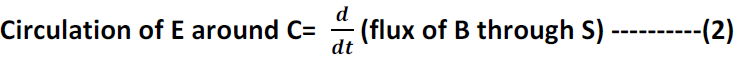We can complete laws of electromagnetic field by writing two corresponding equations for magnetic field B.

Flux of B through any closed surface = 0 -----------(3)

For and surface bounded by curve ‘C’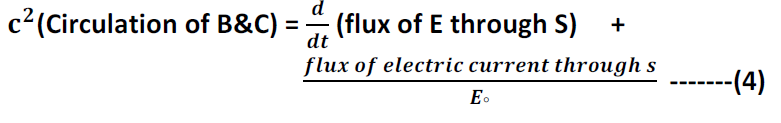Constant  'c' appears in above equation because (“magnetism is in reality a relativistic effect of electricity”).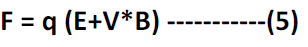The force on charge in general in electromagnetism is given as follows –

The second part of above equation can be demonstrated by passing a current through a wire which hangs above bar magnet

The wire will move when a current is turned on because of force
“F = qv*b”. When a current exists, the charges inside wire are moving, so they have a velocity ‘V’ and magnetic field from magnet exerts a force on them, which results in pushing wire sideways.
When the wire is pushed to left, we would expect that magnet must fell push to right to conserve momentum. Although the force is too small to make movement of bar magnet visible, a more sensitively supported magnet, like a compass needle, we will show movement.

How does the wire push on magnet?
The current in wire produces a magnetic field of its own that exerts a force on magnet. According to last term in equation (4), a current results in circulation of ‘B’ in this case lines of B are loops around wire as shown in figure. This ‘B’ is responsible for force on magnet.
Equation (4) tells us that for a fixed current through wire the circulation of ‘B’ is same for any curve that surrounds the wire.

For curves say circles that are farther away from wire, the circumference is larger, so tangential component of ‘B’ must decrease.

We have said that a current through a wire produces a magnet field, and that when there is a magnetic field present there is a force on a wire carrying a current.

Then we should also expect that if we make a magnetic field with a current in one wire, it should exert a force on another wire which also carries a current. When current are in same direction they attract each other.Electrical currents as well as magnets make magnetic fields.

But what is a magnet anyway
If magnetic fields are produced by moving charges, is it is not possible that magnetic fields from a piece of iron is really the result of current.
We can replace bar magnet of our experiment with a coil of wire carrying current. So a piece of iron acts as though it contains a perpetual circulating current. We can in fact understand magnets in terms of permanent currents in atoms of iron.

Where do these currents come from?
One possibility would be from motion of electrons in atomic orbits. Actually that is not case for iron, although it is for some materials. It is due to spin of electron and it is this current that gives magnetic field in iron.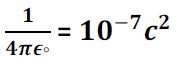All the electromagnetism is contained in Maxwell equations.

Maxwell Equations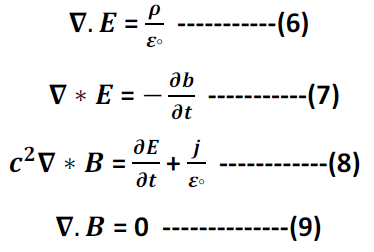Now consider the ‘static’ case. All charges are permanently fixed in space or if they do not move, they move as a steady flow in a circuit.
In these circumstances, all of the terms in the Maxwell equations which are time derivatives of field are zero. So Maxwell equations becomes

Electrostatics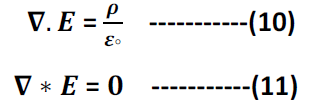Magnetostatics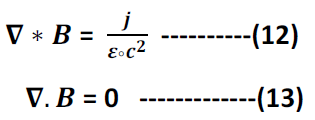This means that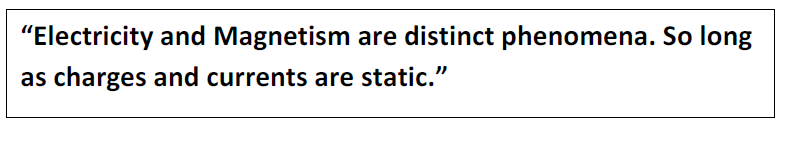The interdependence of E & B does not appear until there are changes in charges or currents, as when a condenser is charged or a magnet moved.
Only when there are sufficiently rapid changes, so that the time derivatives in Maxwell equations become significant, will E & B depend on each other.
Electrostatics is a clean example of vector field with zero curl and a given divergence.
Magnetostatics is a neat example of a field with zero divergence and a given curl.

How to find electric field at a point ‘P’ due to a charge distribution?

The electric field ‘E’ at point , from a charge distribution, is obtained from an integral over the distribution (shown in following figure).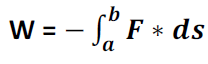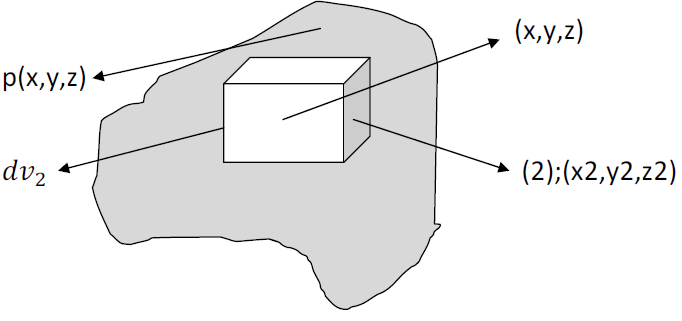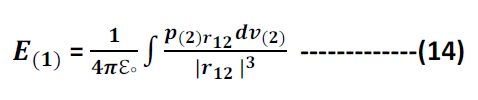The work done against the electric forces in carrying a charge along some path in the negative of component of electric force in direction of motion, integrated along the path. If we carry a charge from point ‘a’ to point ‘b’ then

F  = Electric force on charge at each point.
Ds = Differential vector displacement along path